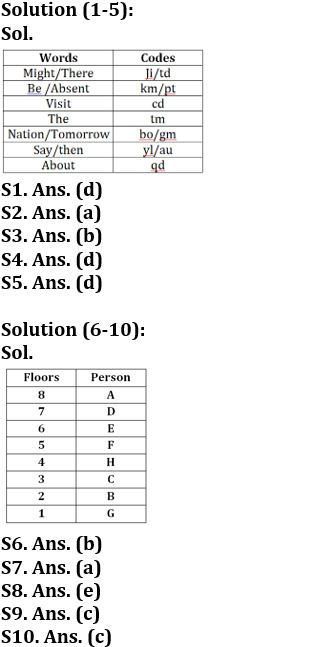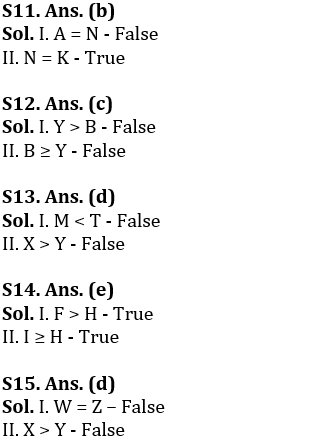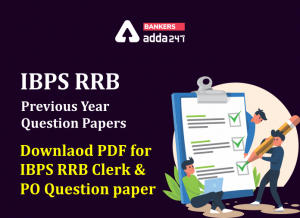Latest Banking jobs   »

# Reasoning Ability Quiz For IBPS RRB PO Clerk Prelims 2022- 19th July

Directions (1-5): Study the following data carefully and answer the questions accordingly.
In a certain code language,
“Might be absent there” is coded as “ji km pt td”
“Visit be absent the” is coded as “tm pt km cd”
“Nation tomorrow say then” is coded as “yl au bo gm”
“Then say the about” is coded as “tm qd au yl”

Q1. What is the code for “absent” in the given code language?
(a) ji
(b) pt
(c) km
(d) Can’t be determined
(e) None of these

Q2. What is the code for “the” in the given code language?
(a) tm
(b) pt
(c) cd
(d) km
(e) None of these

Q3. What is the code for “about” in the given code language?
(a) au
(b) qd
(c) yl
(d) tm
(e) None of these

Q4. Which of the following words is coded as “cd”?
(a) The
(b) Absent
(c) Be
(d) Visit
(e) None of these

Q5. What may be the possible code for “might” in the given code language?
(a) ji
(b) td
(c) km
(d) Either ‘ji’ or ‘td’
(e) None of these

Directions (6-10): Read the following information carefully to answer the questions give below.
Eight persons A, B, C, D, E, F, G and H live in an 8-floor building but not necessarily in the same order. The lowermost floor is numbered as 1, floor just above 1st floor is numbered 2 and so on till the topmost floor is numbered as 8.
Five persons live between A and B. G lives below B. Only three persons live between G and F. As many persons live between A and F as between B and F. More than three persons live between D and B. H lives immediately above C. E does not live on the 4th floor.

Q6. How many persons live between H and A?
(a) 2
(b) 3
(c) 4
(d) 5
(e) None of these

Q7. If all the persons live according to the alphabetical order from top to bottom, then position of how many persons will not change?
(a) 1
(b) 2
(c) 3
(d) None
(e) None of these

Q8. Four of the following five pairs are alike in a certain manner and hence form a group. Find the one that does not belong to that group?
(a) D, E
(b) C, B
(c) F, H
(d) B, G
(e) E, H

Q9. Who among the following lives just below E?
(a) D
(b) H
(c) F
(d) B
(e) None of these

Q10. If A is related to G and D is related to B, then F is related to whom in the same pattern?
(a) C
(b) E
(c) H
(d) Either C or H
(e) None of these

Directions (11-15): In the following questions assuming the given statement to be true, find which of the conclusion(s) among given conclusions is/are definitely true and then give your answers accordingly.

Q11. Statements: A > G ≥ H ≤ I, F > K = N, N = M
Conclusions: I. A = N
II. N = K
(a) Only I is true.
(b) Only II is true.
(c) Either I or II is true.
(d) Neither I nor II is true.
(e) Both I and II are true.

Q12. Statements: A > B ≥ C ≤ I, A > Y = L, L = M
Conclusions: I. Y > B
II. B ≥ Y
(a) Only I is true.
(b) Only II is true.
(c) Either I or II is true.
(d) Neither I nor II is true.
(e) Both I and II are true.

Q13. Statements: W > F ≥ Y ≤ T, F > X = L, L = M
Conclusions: I. M < T
II. X > Y
(a) Only I is true.
(b) Only II is true.
(c) Either I or II is true.
(d) Neither I nor II is true.
(e) Both I and II are true.

Q14. Statements: F > G ≥ H ≤ I, F > K = L
Conclusions: I. F > H
II. I ≥ H
(a) Only I is true.
(b) Only II is true.
(c) Either I or II is true.
(d) Neither I nor II is true.
(e) Both I and II are true.

Q15. Statements: W > X ≥ Y ≤ Z, F > K = N, N = M
Conclusions: I. W = Z
II. X > Y
(a) Only I is true.
(b) Only II is true.
(c) Either I or II is true.
(d) Neither I nor II is true.
(e) Both I and II are true.

Solutions#### Congratulations!Incorrect details? Fill the form again here

•Reasoning Ability Quiz For IBPS RRB PO C...
•Quantitative Aptitude Quiz For IBPS RRB ...
•Reasoning Ability Quiz For IBPS RRB PO C...
•Quantitative Aptitude Quiz For IBPS RRB ...
•IBPS RRB Previous Year Question Paper PD...
•Quantitative Aptitude Quiz For IBPS RRB ...